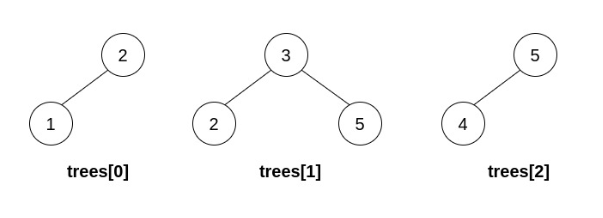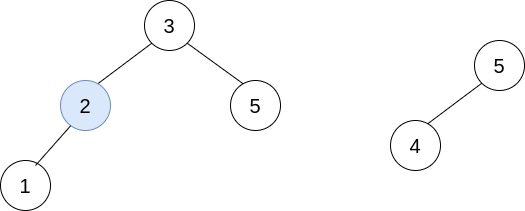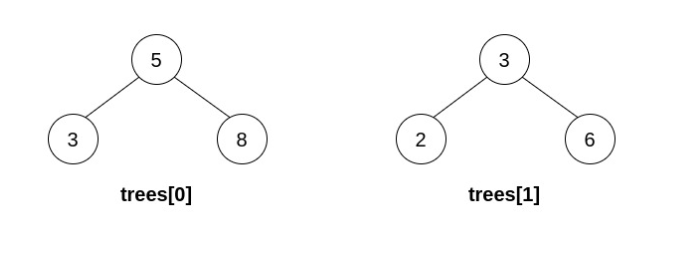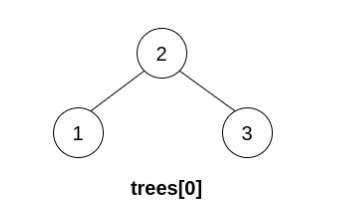# 合并多棵二叉搜索树

## 1932. 合并多棵二叉搜索树 (Hard)

• 选择两个 不同的 下标 `i``j` ，要求满足在 `trees[i]` 中的某个 叶节点 的值等于 `trees[j]`根节点的值
• 用 `trees[j]` 替换 `trees[i]` 中的那个叶节点。
• `trees` 中移除 `trees[j]`

• 任意节点的左子树中的值都 严格小于 此节点的值。
• 任意节点的右子树中的值都 严格大于 此节点的值。```输入：trees = [[2,1],[3,2,5],[5,4]]```输入：trees = [[5,3,8],[3,2,6]]``````输入：trees = [[5,4],]

``````输入：trees = [[2,1,3]]

```

• `n == trees.length`
• `1 <= n <= 5 * 104`
• 每棵树中节点数目在范围 `[1, 3]` 内。
• 输入数据的每个节点可能有子节点但不存在子节点的子节点
• `trees` 中不存在两棵树根节点值相同的情况。
• 输入中的所有树都是 有效的二叉树搜索树
• `1 <= TreeNode.val <= 5 * 104`.

### 相关话题

[] [深度优先搜索] [哈希表] [二分查找] [二叉树]